Ex 14.2

Chapter 14 Class 11 Probability
Serial order wise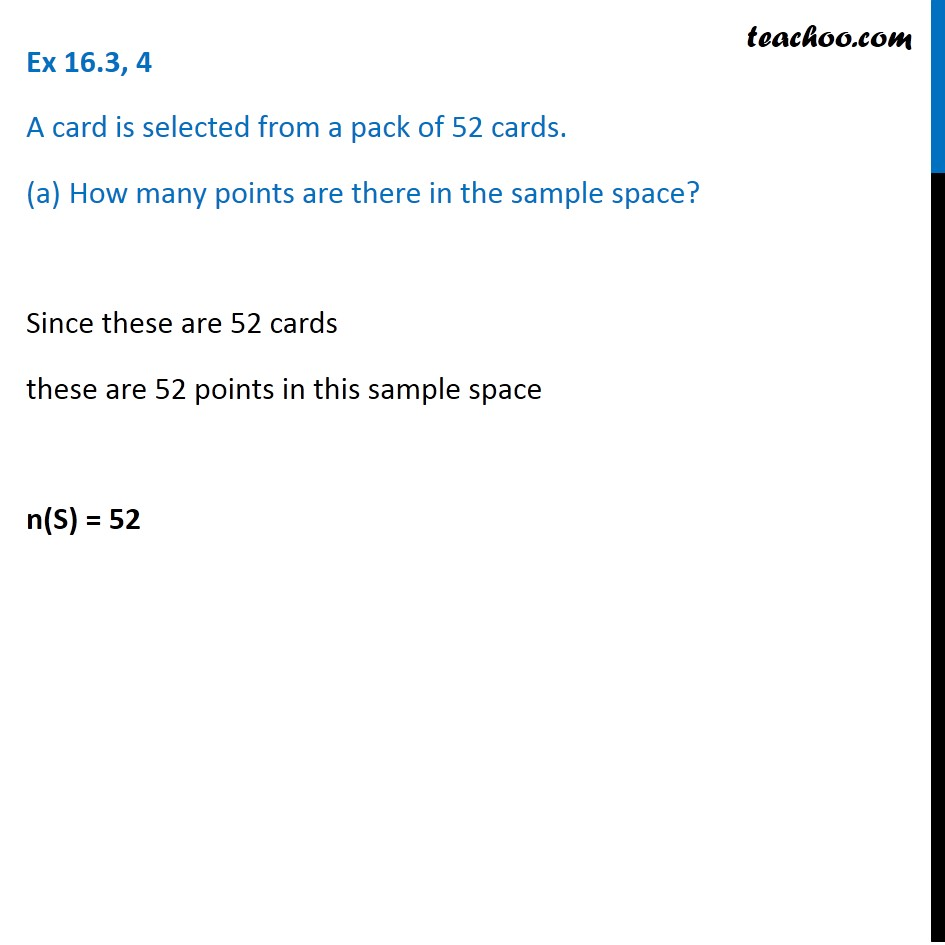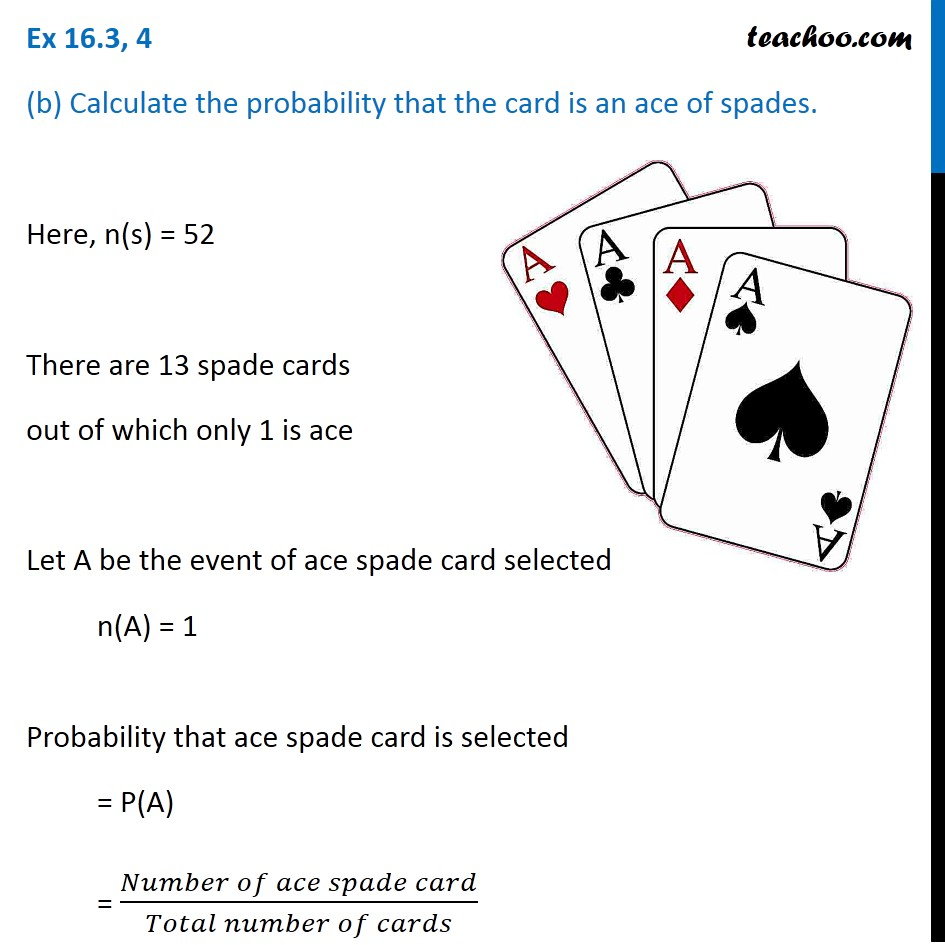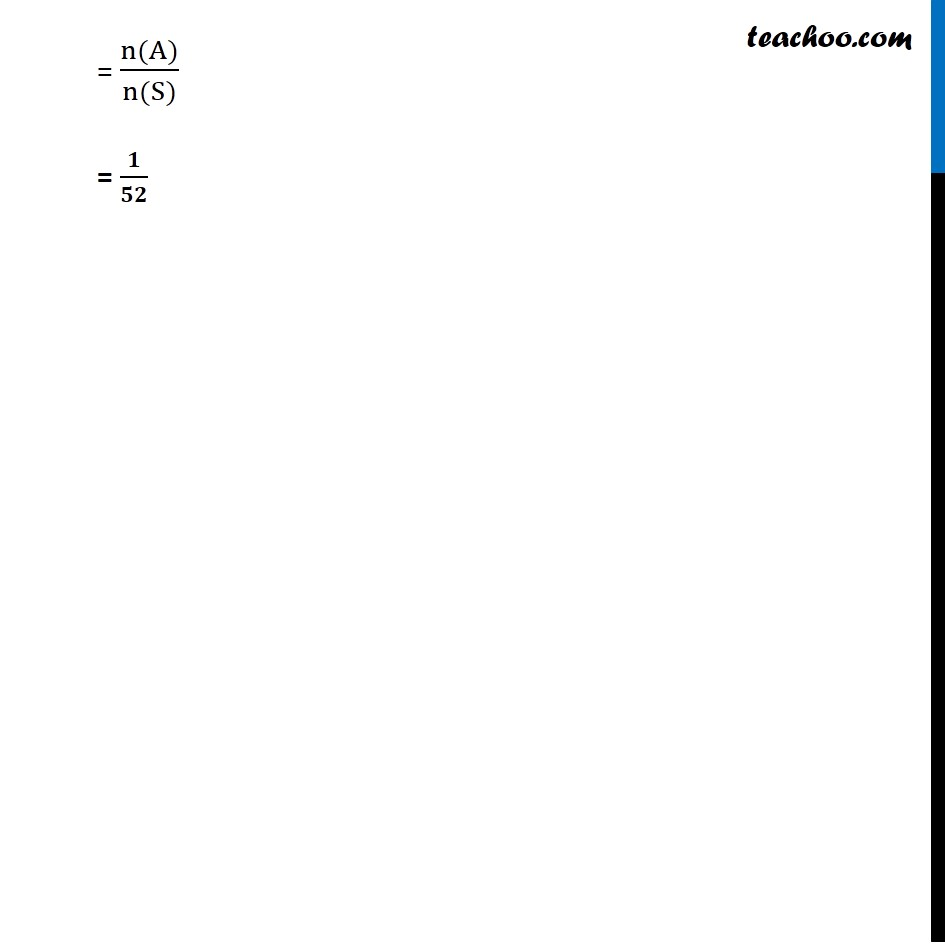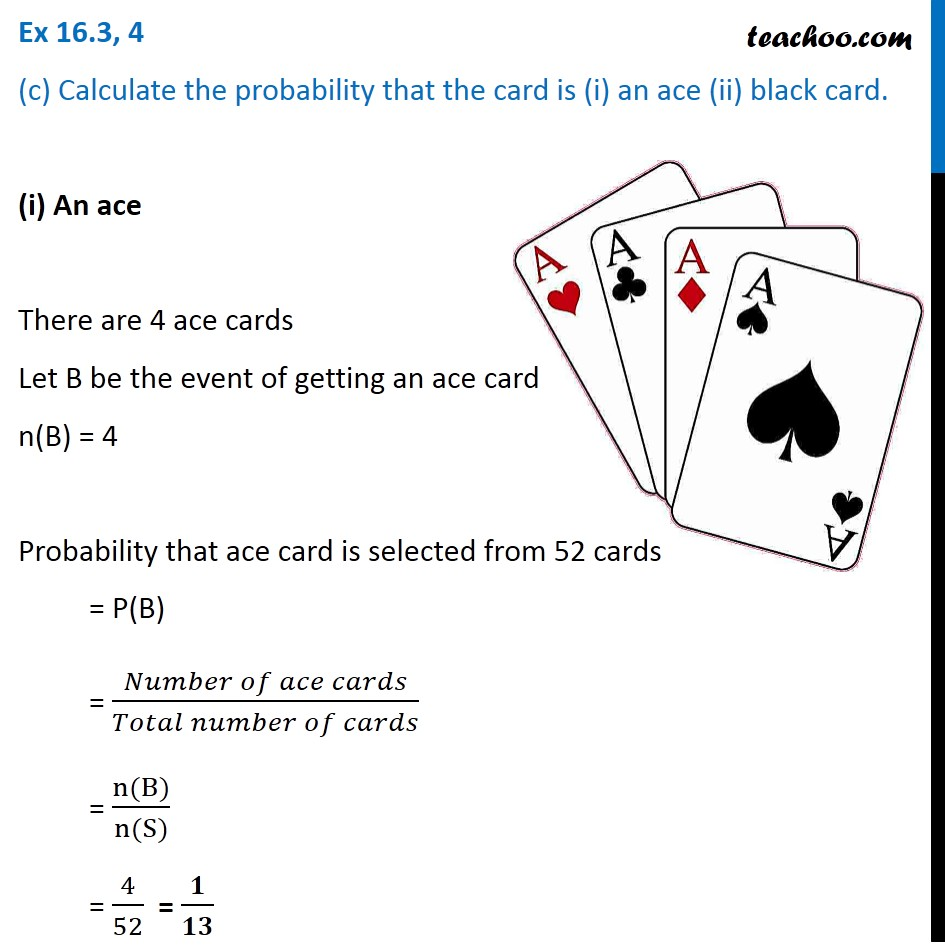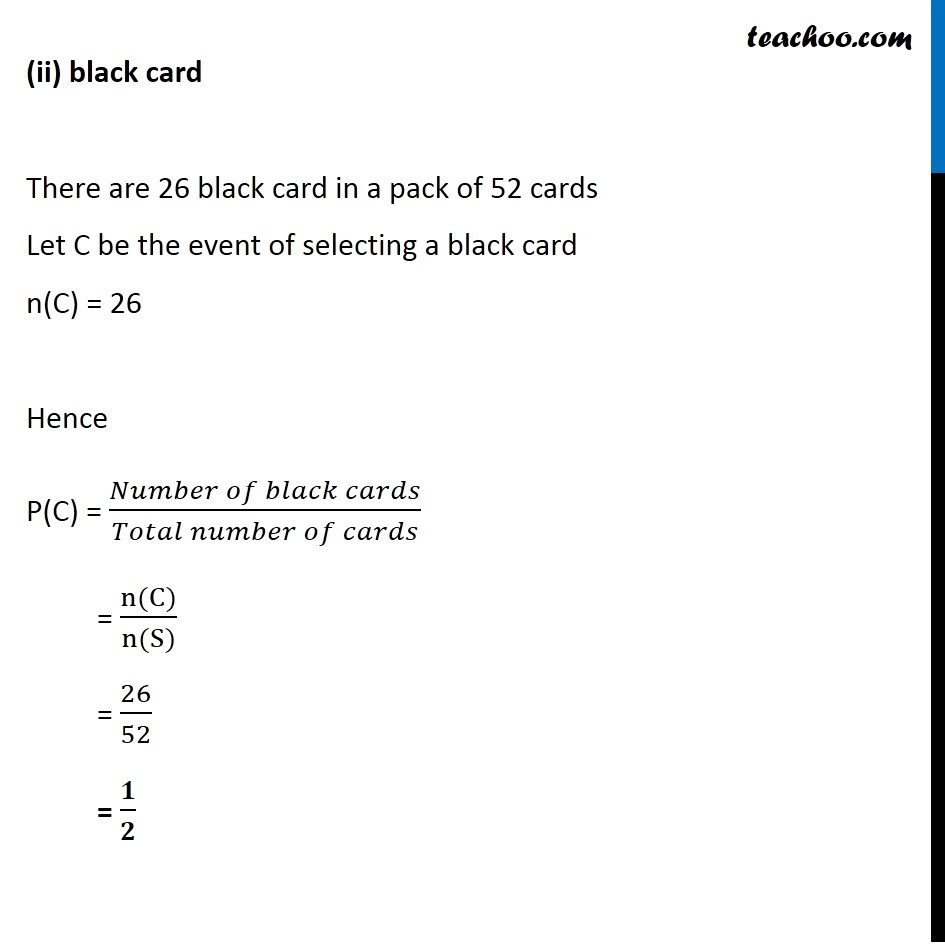Learn in your speed, with individual attention - Teachoo Maths 1-on-1 Class

### Transcript

Ex 14.2, 4 A card is selected from a pack of 52 cards. How many points are there in the sample space? Since these are 52 cards these are 52 points in this sample space n(S) = 52 Ex 14.2, 4 (b) Calculate the probability that the card is an ace of spades. Here, n(s) = 52 There are 13 spade cards out of which only 1 is ace Let A be the event of ace spade card selected n(A) = 1 Probability that ace spade card is selected = P(A) = (𝑁𝑢𝑚𝑏𝑒𝑟 𝑜𝑓 𝑎𝑐𝑒 𝑠𝑝𝑎𝑑𝑒 𝑐𝑎𝑟𝑑)/(𝑇𝑜𝑡𝑎𝑙 𝑛𝑢𝑚𝑏𝑒𝑟 𝑜𝑓 𝑐𝑎𝑟𝑑𝑠) = (n(A))/(n(S)) = 𝟏/𝟓𝟐 Ex 14.2, 4 (c) Calculate the probability that the card is (i) an ace (ii) black card. (i) An ace There are 4 ace cards Let B be the event of getting an ace card n(B) = 4 Probability that ace card is selected from 52 cards = P(B) = (𝑁𝑢𝑚𝑏𝑒𝑟 𝑜𝑓 𝑎𝑐𝑒 𝑐𝑎𝑟𝑑𝑠)/(𝑇𝑜𝑡𝑎𝑙 𝑛𝑢𝑚𝑏𝑒𝑟 𝑜𝑓 𝑐𝑎𝑟𝑑𝑠) = (n(B))/(n(S)) = 4/52 = 𝟏/𝟏𝟑 (ii) black card There are 26 black card in a pack of 52 cards Let C be the event of selecting a black card n(C) = 26 Hence P(C) = (𝑁𝑢𝑚𝑏𝑒𝑟 𝑜𝑓 𝑏𝑙𝑎𝑐𝑘 𝑐𝑎𝑟𝑑𝑠)/(𝑇𝑜𝑡𝑎𝑙 𝑛𝑢𝑚𝑏𝑒𝑟 𝑜𝑓 𝑐𝑎𝑟𝑑𝑠) = (n(C))/(n(S)) = 26/52 = 𝟏/𝟐